## Problem Solving Lesson 4: Multiplication and division of one-variable polynomials (C7 Math 7 Horizons) – Math Book

Solving Exercises Lesson 4: Multiplication and division of one-variable polynomials (C7 Math 7 Horizon)
==========

### Solution 1 page 40 Math textbook 7 Creative horizon volume 2

Perform multiplication.

a) $$(4x – 3)(x + 2)$$

b) $$(5x + 2)( – {x^2} + 3x + 1)$$

c) $$(2{x^2} – 7x + 4)( – 3{x^2} + 6x + 5)$$

Solution method

Apply the rules for multiplying polynomials (distributions).

Detailed explanation

a) $$\begin{array}{l}(4x – 3)(x + 2) = 4x(x + 2) – 3(x + 2)\\ = 4{x^2} + 8x – 3x – 6\end{array}$$

$$= 4{x^2} + 5x – 6$$

b) $$(5x + 2)( – {x^2} + 3x + 1)$$

$$= 5x( – {x^2} + 3x + 1) + 2( – {x^2} + 3x + 1)$$

$$= – 5{x^3} + 15{x^2} + 5x – 2{x^2} + 6x + 2$$

$$= – 5{x^3} + 13{x^2} + 11x + 2$$

c) $$(2{x^2} – 7x + 4)( – 3{x^2} + 6x + 5)$$

$$= 2{x^2}( – 3{x^2} + 6x + 5) – 7x( – 3{x^2} + 6x + 5) + 4( – 3{x^2} + 6x + 5)$$

$$= 2{x^2}( – 3{x^2}) + 2{x^2}.6x + 2{x^2}.5 + 7x.3{x^2} – 7x.6x – 7x.5 + 4( – 3{x^2}) + 4.6x + 4.5$$

$$= – 6{x^4} + 33{x^3} – 44{x^2} – 11x + 20$$

### Solution 2 page 40 Math textbook 7 Creative horizon volume 2

Given two rectangles as shown in Figure 4. Find the polynomial in terms of the variable x representing the area of ​​the blue shaded part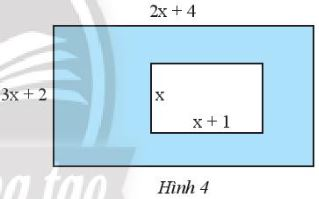Solution method

We calculate the area of ​​the large rectangle

We calculate the area of ​​the small rectangle

Subtract the large area from the small area to find the area to find

Detailed explanation

The area of ​​the large rectangle is: $$(2x + 4)(3x + 2) = 2x + 4(3x + 2) = 6{x^2} + 4x + 12x + 8 = 6{x^2 } + 16x + 8$$

The area of ​​the small rectangle is : $$x(x + 1) = {x^2} + x$$

The area to find is: $$6{x^2} + 16x + 8 – {x^2} – x$$$$= 5{x^2} + 15x + 8$$

### Solve problems 3 pages 40 Math textbook 7 Creative horizon volume 2

Perform division.

a) $$(8{x^6} – 4{x^5} + 12{x^4} – 20{x^3}):4{x^3}$$

b) $$(2{x^2} – 5x + 3):(2x – 3)$$

Solution method

Perform multiplication and division of polynomials by setting the calculator.

Note: Sort polynomials in descending order of powers

Detailed explanation

a) $$(8{x^6} – 4{x^5} + 12{x^4} – 20{x^3}):4{x^3}$$

$$= (8{x^6}:4{x^3}) – (4{x^5}:4{x^3}) + (12{x^4}:4{x^3}) – (20{x^3}:4{x^3})$$

$$= 2{x^2} – {x^2} + 3x – 5$$

b)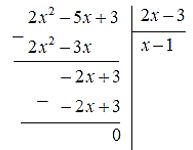So $$(2{x^2} – 5x + 3):(2x – 3)= x – 1$$

### Solve problems 4 pages 40 Math textbook 7 Creative horizon volume 2

Perform division.

a) $$(4{x^2} – 5):(x – 2)$$

b) $$(3{x^3} – 7x + 2):(2{x^2} – 3)$$

Solution method

Use learned rules to divide polynomials

It is recommended to arrange the polynomial in descending power to make it easy to calculate

Detailed explanation

a) $$(4{x^2} – 5):(x – 2) = \dfrac{{4{x^2} – 5}}{{x – 2}} = 4x + 8 + \dfrac{ {11}}{{x – 2}}$$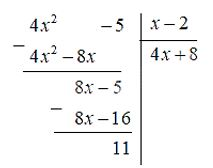So $$(4{x^2} – 5):(x – 2)= 4x + 8 + \dfrac{{11}}{{x – 2}}$$

b) $$(3{x^3} – 7x + 2):(2{x^2} – 3) = \dfrac{{3{x^3} – 7x + 2}}{{2{x^ 2} – 3}}$$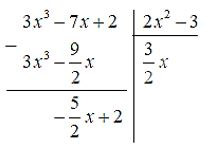So $$(3{x^3} – 7x + 2):(2{x^2} – 3)= \dfrac{3}{2}x – \dfrac{{\dfrac{5}{2}x + 2}}{{2{x^2} – 3}}$$

### Solve problems 5 pages 40 Math textbook 7 Creative horizon volume 2

Calculate the length of a rectangle whose area is equal to $$(4{y^2} + 4y – 3)$$$$c{m^2}$$ and width is equal to (2y – 1) cm.

Solution method

Use the formula to calculate the area of ​​a rectangle

Apply the polynomial division rule and arrange the polynomials in descending order of powers

Detailed explanation

Area of ​​rectangle = length. width

$$\Rightarrow (4{y^2} + 4y – 3):(2y – 1) = \dfrac{{4{y^2} + 4y – 3}}{{2y – 1}}$$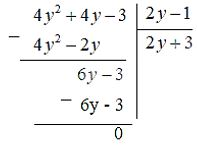So the length of the rectangle is: 2y + 3 cm

### Solving problems 6 pages 40 Math textbook 7 Creative horizon volume 2

Given a rectangular box with volume equal to ($$3{x^3} + 8{x^2} – 45x – 50$$) $$c{m^3}$$, length equal to (x + 5 ) cm and the height is equal to (x + 1) cm. Calculate the width of that rectangular box.

Solution method

Use the formula to calculate the volume of a rectangular box V=Sh

Apply the rule of division by two polynomials

Detailed explanation

The volume of a rectangular box is equal to the product of the height and the area of ​​the base

So width of rectangle = volume : ( height . length )

The area of ​​the base is $$(x + 5)(x + 1) = {x^2} + 6x + 5$$

Substituting the numbers in the problem for the above formula we get:

$$= \dfrac{{3{x^3} + 8{x^2} – 45x – 50}}{{(x + 5)(x + 1) = {x^2} + 6x + 5}} = \dfrac{{3{x^3} + 8{x^2} – 45x – 50}}{{{x^2} + 6x + 5}}$$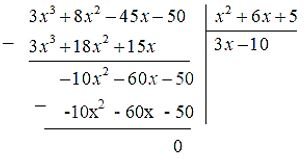So the length of the rectangular box is 3x – 10 cm